Open in App
Not now

# Comparison Operators in SQL

• Difficulty Level : Expert
• Last Updated : 14 Nov, 2021

In SQL, there are six comparison operators available which help us run queries to perform various operations. We will use the WHERE command along with the conditional operator to achieve this in SQL. For this article, we will be using the Microsoft SQL Server as our database.

Syntax:

```SELECT * FROM TABLE_NAME WHERE
ATTRIBUTE CONDITION_OPERATOR GIVEN_VALUE;```

Step 1: Create a Database. For this use the below command to create a database named GeeksForGeeks.

Query:

`CREATE DATABASE GeeksForGeeks`

Output: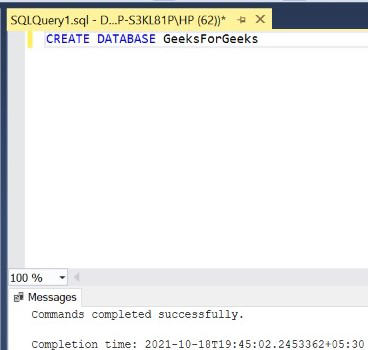Step 2: Use the GeeksForGeeks database. For this use the below command.

Query:

`USE GeeksForGeeks`

Output: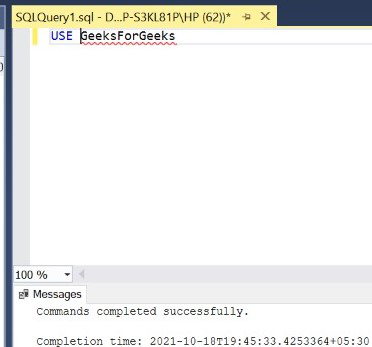Step 3: Create a table MATHS inside the database GeeksForGeeks. This table has 3 columns namely ROLL_NUMBER, S_NAME and MARKS containing roll number, student name, and marks obtained in math’s subject by various students.

Query:

```CREATE TABLE MATHS(
ROLL_NUMBER INT,
S_NAME VARCHAR(10),
MARKS INT);```

Output: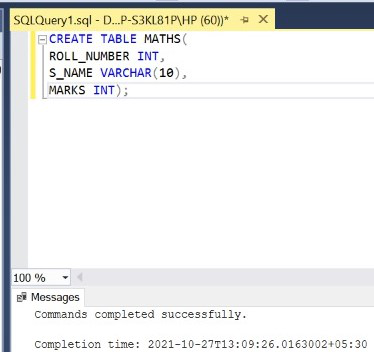Step 4: Display the structure of the MATHS table.

Query:

`EXEC SP_COLUMNS 'MATHS';`

Output: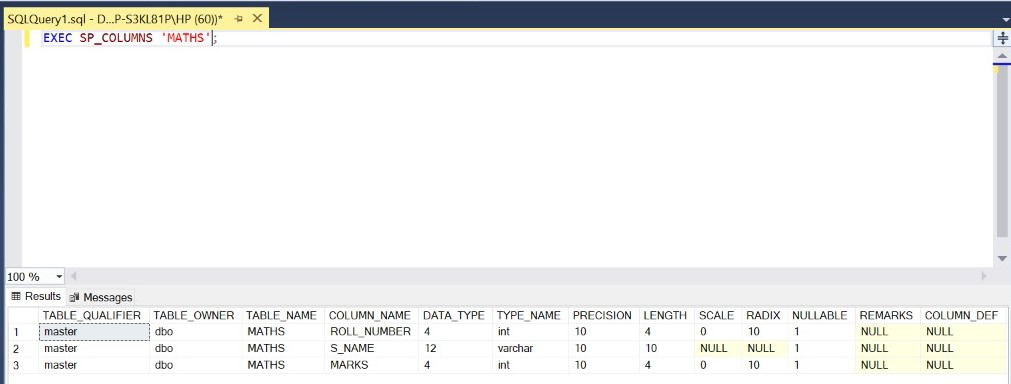Step 5: Insert 10 rows into the MATHS table.

Query:

```INSERT INTO MATHS VALUES(1,'ABHI',70);
INSERT INTO MATHS VALUES(2,'RAVI',80);
INSERT INTO MATHS VALUES(3,'ARJUN',90);
INSERT INTO MATHS VALUES(4,'SAM',100);
INSERT INTO MATHS VALUES(5,'MOHAN',50);
INSERT INTO MATHS VALUES(6,'ROHAN',10);
INSERT INTO MATHS VALUES(7,'ROCKY',20);
INSERT INTO MATHS VALUES(8,'AYUSH',40);
INSERT INTO MATHS VALUES(9,'NEHA',30);
INSERT INTO MATHS VALUES(10,'KRITI',60);```

Output: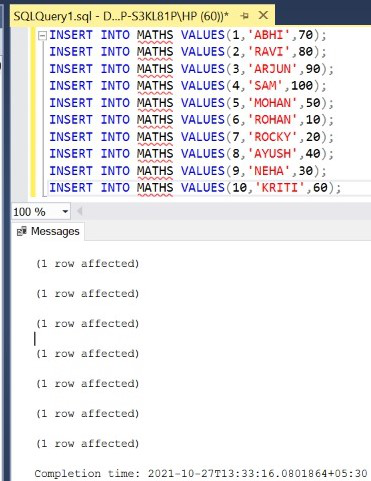Step 6: Display all the rows of the MATHS table.

Query:

`SELECT * FROM MATHS;`

Output: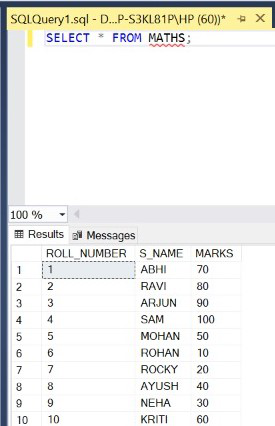Demonstration of various Comparison Operators in SQL:

• Equal to (=) Operator: It returns the rows/tuples which have the value of the attribute equal to the given value.

Query:

`SELECT * FROM MATHS WHERE MARKS=50;`

Output: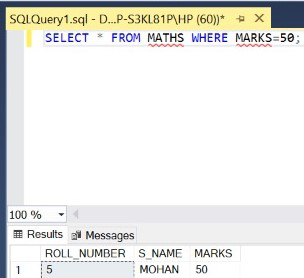• Greater than (>) Operator: It returns the rows/tuples which have the value of the attribute greater than the given value.

Query:

`SELECT * FROM MATHS WHERE MARKS>60;`

Output: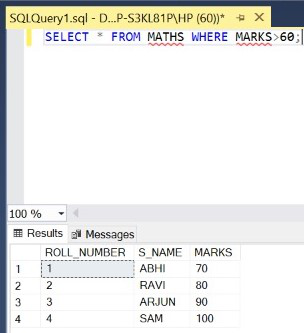• Less than (<) Operator: It returns the rows/tuples which have the value of the attribute lesser than the given value.

Query:

`SELECT * FROM MATHS WHERE MARKS<40;`

Output: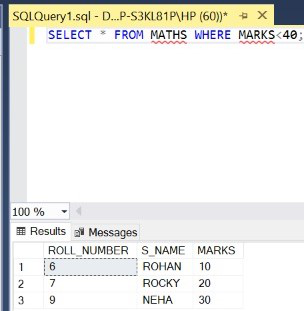• Greater than or equal to (>=) Operator: It returns the rows/tuples which have the value of the attribute greater or equal to the given value.

Query:

`SELECT * FROM MATHS WHERE MARKS>=80;`

Output: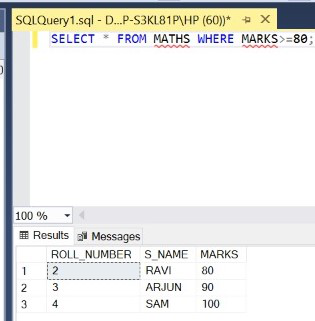• Less than or equal to (<=) Operator: It returns the rows/tuples which have the value of the attribute lesser or equal to the given value.

Query:

`SELECT * FROM MATHS WHERE MARKS<=30;`

Output: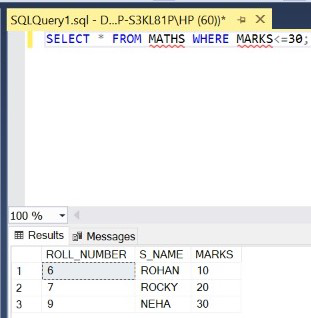• Not equal to (<>) Operator: It returns the rows/tuples which have the value of the attribute not equal to the given value.

Query:

`SELECT * FROM MATHS WHERE MARKS<>70;`

Output: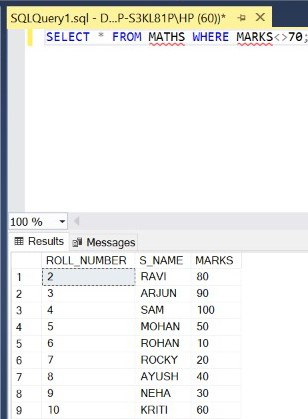My Personal Notes arrow_drop_up